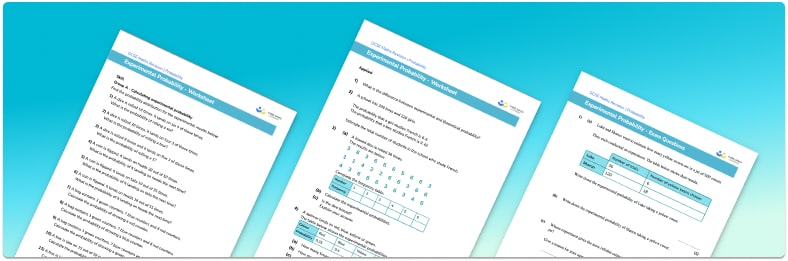# Experimental Probability Worksheet• Section 1 of the experimental probability worksheet contains 20+ skills-based experimental probability questions, in 3 groups to support differentiation
• Section 2 contains 3 applied experimental probability questions with a mix of worded problems and deeper problem solving questions
• Section 3 contains 4 foundation and higher level GCSE exam style experimental probability questions
• Answers and a mark scheme for all experimental probability questions are provided
• Questions follow variation theory with plenty of opportunities for students to work independently at their own level
• All questions created by fully qualified expert secondary maths teachers

Suitable for GCSE maths revision for AQA, OCR and Edexcel exam boards

• This field is for validation purposes and should be left unchanged.

You can unsubscribe at any time (each email we send will contain an easy way to unsubscribe). To find out more about how we use your data, see our privacy policy.

### Experimental probability at a glance

Experimental probability, also called relative frequency, is the probability of an event occurring based on previous events. This is useful when we don’t know the theoretical probability of an event occurring. For example, a biased spinner has three possible outcomes: red, green, and blue. We do not know the probability of each outcome but when we spin the spinner 100 times, it lands on green 17 times. The experimental probability of landing on green is 17 out of 100.

As we increase the number of trials in our experiment, the accuracy of the theoretical probability improves, and the theoretical and experimental probabilities become more similar.

Looking forward, students can then progress to additional probability worksheets, for example the Venn diagram worksheet, the mutually exclusive events worksheet, or the probability tree diagram worksheet.For more teaching and learning support on probability our GCSE maths lessons provide step by step support for all GCSE maths concepts.

## Do you have KS4 students who need more focused attention to succeed at GCSE?There will be students in your class who require individual attention to help them succeed in their maths GCSEs. In a class of 30, it’s not always easy to provide.

Help your students feel confident with exam-style questions and the strategies they’ll need to answer them correctly with our dedicated GCSE maths revision programme.

Lessons are selected to provide support where each student needs it most, and specially-trained GCSE maths tutors adapt the pitch and pace of each lesson. This ensures a personalised revision programme that raises grades and boosts confidence.

Find out more